### Home > CALC > Chapter 9 > Lesson 9.4.1 > Problem9-113

9-113.
1. No calculator! Evaluate the following limits. Homework Help ✎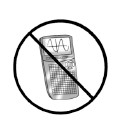1.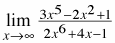2.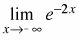3.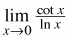4.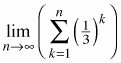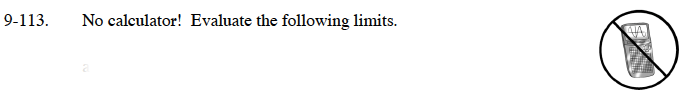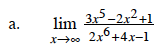What is the dominant term in the numerator? What is the dominant term in the denominator? How do these terms compare?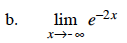Graph y = e–2x. What happens to the graph as x → –∞?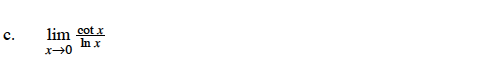l'Hôpital's Rule can be used here. Or, you can graph the function.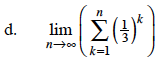This is the geometric series:

$\frac{1}{3}+\frac{1}{9}+\frac{1}{27}+...$